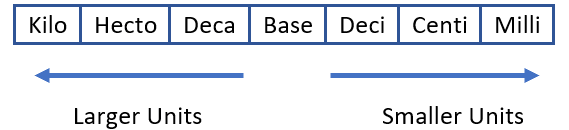# Math Worksheets Land

Math Worksheets For All Ages

# Math Worksheets Land

Math Worksheets For All Ages

# Relative Sizes of Measurement Units Worksheets

Having the ability to convert various measures throughout the metric system is a structural skill that all students will practice over the course of many different years. I find that students have a general understanding as they proceed from one grade level to the next, but they are in a constant need of remediation. The only time it truly sets in is when students are actively using this skill in a laboratory setting regularly. That is because it is easy to forget a paper and pencil version of this skill, but once you break out the tools and get your hands on making these measures it become concrete. This series of worksheets and lessons has students learn relative sizes of units of measure.

### Aligned Standard: Grade 4 Fractions - 4.MD.1

• Answer Keys - These are for all the unlocked materials above.

### Homework Sheets

I mixed short and sweet word problems with your standard conversion problems.

### Practice Worksheets

You will see every type of format that I have ever seen these types of problems posed to students for this level.

### Math Skill Quizzes

The quizzes all stick with the standard unit conversion format. No word problems here.

• Quiz 1 - 720 seconds = ______minutes
• Quiz 2 - 80 hectometers =__kilometers
• Quiz 3 - 5 liters = _______milliliters

### Tips for Converting Units of Measure

When we are working in many different fields of study it is often extremely important to properly communicate the exact amount of a substance that we are working with or observing. There are many different types of units of measure and tools that are calibrated to different standards. In order to work with all these different measures and tools we must be able to convert measures between different units as needed.

The metric scale is grounded on the same base ten system as the place value system. Here is look at the basic metric scale:The base is the fundamental unit of measure. In the case of length, a meter is the base metric unit used. As we can see from the chart, a kilometer is 3 positions larger than a meter. That would mean that a kilometer is 1,000 (10 x 10 x 10 = 1,000) times greater. When we are working with measures within the metric system it is a simple matter of identifying where you are currently at and where you need to convert to. Movements to the left are larger by 10 times for each move and movement to the right are 10 times smaller.

## Working with Compound Units of Measure

There are many times where converting units of measure is simple such as between meters and kilometers. That is increasing the scale a 1,000 times over. Converting compound measures of units can sometimes be a nightmare; however, there are a few tips and tricks that can be used to convert one unit to another safely. For example, we can convert km/h (kilometers per hour) to m/s (meters per second) like this:

One kilometer has 1000 meters and one hour has 3600 seconds, so the km/h is:

1000/3600 = 0.277 m/s

In order to know how to make it 1000/3600 and not write it, 3600/1000 is doing the conversion as fractions. Know when to divide and when to multiply.

Aside from knowing how many km is equal to how many meters, the trickiest part of converting is knowing when you should be dividing the number or multiplying. For example, in our previous example, we understood that 1 km equals 1000 m but how to get the two units converted into the other. Here is how you do it:

Remember, multiply gives us more, and division gives us less. Therefore, When we move from a bigger unit to the smaller one, we multiply. When we move from a smaller unit to the bigger ones, we divide.

Unlock all the answers, worksheets, homework, tests and more!
Save Tons of Time! Make My Life Easier Now

## Thanks and Don't Forget To Tell Your Friends!

I would appreciate everyone letting me know if you find any errors. I'm getting a little older these days and my eyes are going. Please contact me, to let me know. I'll fix it ASAP.